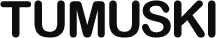# Simple Interfaciness in JavaScript

Posted at 3:59 pm on October 16th, 2009

## Filed in:

Here’s a script (iFace.js) that provides some simple interface-like behavior in JavaScript by asserting that a given constructor must produce objects that have certain members.

``````var greeterIF = iFace('greeterIF', [
'hello',
'goodbye'
]);``````

(The first argument, `'greeterIF'` is for the potential error messages that might occur, and it can be any string. It doesn’t have to be the same as the variable that gets the result, but it will aid your effort in finding the error.)

Now `greeterIF` is a function that we can pass constructors through before they actually construct anything.

``````var EnglishGreeter, GermanGreeter, CanadianGreeter;

EnglishGreeter = greeterIF('EnglishGreeter', function (name) {
this.hello = function () {
return "Hi there, " + name + "!";
};
this.goodbye = function () {
return "Good bye, " + name + "!";
};
});

GermanGreeter = greeterIF('GermanGreeter', function (name) {
this.hello = function () {
return "Wie geht's, " + name + "?";
};
this.goodbye = function () {
return "Auf Wiedersehen, " + name + "!";
};
});

this.hello = function () {
return "How's it goin', eh " + name + "?";
};
});``````

(Regarding the first argument, the same note above applies here as well.)

Notice how the `CanadianGreeter` constructor doesn’t assign a `goodbye` method. This will cause an error, but not until it’s instantiated.

``````var english, german, canadian;

english = new EnglishGreeter("Thomas");
german = new GermanGreeter("Inge");

The first two will instantiate fine, but the third will throw an error.

But what if it’s 3am, and I forget to pass the constructor through the `greeterIF` function?

``````var KiswahiliGreeter, kiswahili;

KiswahiliGreeter = function (name) {
this.hello = function () {
return "Hujambo, " + name + "?";
};
};

kiswahili = new KiswahiliGreeter("Kivuli");``````

This won’t throw an error. That’s where the second part comes in. The function `iFace` returns has a `verify` method that can be used on an object to see if it has all the members it needs.

``````var Game = function () {
var myGreeter;

/* presumably-rad game code removed */

this.setGreeter = function (g) {
greeterIF.verify(g, 'Game.setGreeter');
myGreeter = g;
};
};``````

Now, if we do this, we get an error:

``````var myGame = new Game();
myGame.setGreeter(kiswahili);``````

Constructors can implement more than one interface. Just pass it through one function after another:

``````var adderIF, subtracterIF, BothDoer, myDoer;

]);

subtracterIF = iFace('subtracterIF', [
'subtract'
]);

BothDoer = adderIF(subtracterIF('BothDoer', function (number) {
};
this.subtract = function (subtrahend) {
number -= subtrahend;
};
this.getNumber = function () {
return number;
};
}));

myDoer = new BothDoer(10);``````

However, the `verify` method only works for one interface at a time, because it’s a method of the interface function itself.